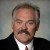# What Is the Correct Manner to Count Defects and Opportunities, Used for Calculating Process Sigma Value?

ByDr. Mikel Harry

As you likely know, the issue of opportunity counting is somewhat illusive. It is laced with many misconceptions. The seeming intuitive nature of a “defect opportunity” runs wider and deeper than what one would naturally expect. To help us better understand this concept (and some of the related pitfalls), we will discuss the classic quality metric called defects per opportunity, or DPO for short. Naturally, we recognize this metric as DPO = D / O, where D is the number of defects and O is the number of defect opportunities.

Every time a defect opportunity is created, it must be assigned a quality condition (0 or1). Of course, this condition is reflected in the numerator term of the DPO metric. In addition, we must account for the original creation of that opportunity by assigning a value of 1 to the denominator term. In this context, every time an opportunity is created, two  independent but related slots are reserved for inclusion in the DPO metric – one slot for the numerator (quality condition) and one slot for the denominator. In this sense, the two slots are married and are, therefore, considered conjugal.

To illustrate, consider a process that is designed to create a certain type of performance opportunity, called O. If that process is cycled C = 5 times, then O = 5 defect opportunities (numerator-denominator pairs) would necessarily be created. We naturally recognize that each opportunity is independently created. For any given pair created, the combinations 0,1 or 1,1 constitute the only possible pair-wise outcomes. Given this, we must necessarily recognize that it is not possible to define four combinations, simply because the denominator term can only be assigned a value of 1 following the creation of its associated opportunity.

Handpicked Content:   Using Core Effectiveness Metrics to Drive Improvement

In furtherance of our example, we will postulate that O = 5 defect opportunities were created and subsequently assessed for conformance to standards, thereby converting their status from passive to active. For the time being, we will say that each of the O = 5 active defect opportunities were determined to be non-conforming and, therefore, defective. Given this, it can now be said that D = 5 defects were realized.

With this in mind, we compute the quantity DPO = D / O = ( 1 + 1 + 1 + 1 + 1 ) / ( 1 + 1 + 1 + 1 + 1 ) = 5 / 5 = 1.00. In this instance, the defect rate would be 100 percent. Given that each active defect opportunity is independent and mutually exclusive, we naturally understand that the defect rate cannot exceed 100 percent. In other words, only one defect can be declared for any given occurrence of an opportunity. Again, this references the conjugal nature of the numerator-denominator pairs that comprise the DPO metric.

Reasoning from the other side, let us now assume that each of the O = 5 active defect opportunities passed the quality examination. In other words, we recognize that each opportunity yielded its performance expectation, thereby establishing the case D = 0. Based on this, we would compute the quantity DPO = D / O = ( 0 + 0 + 0 + 0 + 0 ) / ( 1 + 1 + 1 + 1 + 1 ) = 0 / 5 = 0.00.  In this particular instance, the defect rate would be zero percent.